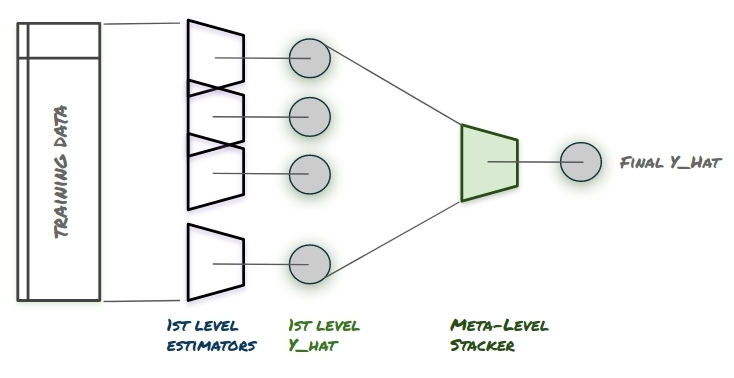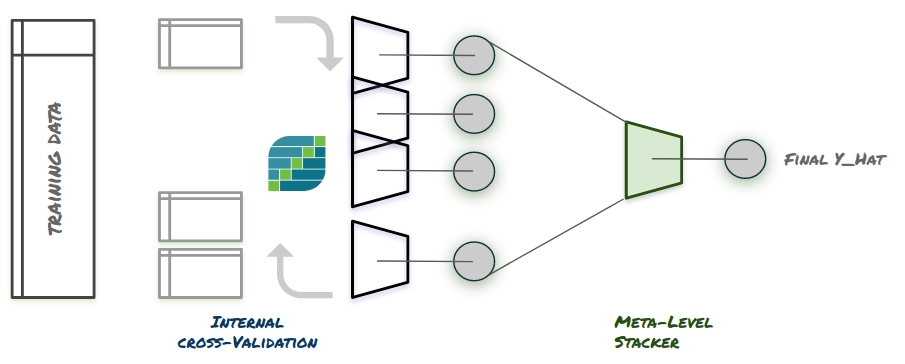# The Stacking Ensemble¶

## Background¶

Stacking (sometimes called stacked generalization or bagging) is an ensemble meta-algorithm that attempts to improve a model’s predictive power by harnessing multiple models (perferably different in nature) to a unified pipeline.

The Stacking method is a very general name that is sometimes used to describe different methods to crete the unfied pipeline. Here, we focus on a Stacking ensemble which uses the multiple models predict the target, while unifing them using a meta-level regressor - which learns how to annotate proper weights to the predictions of the models under it.

A simpler type of Stacking might have been to average the predictions of the different models (similar to Random Forest, but perhaps without the limitation of a single-type model).

In true Stacking the “stacker” or the meta-level regressor can also perform learning, where models which are proven to be less efficient in predicting the data are provided lower weight in the final prediction.high-level description of the stacking ensemble

## Getting it Wrong¶

The major problem in creating a proper Stacking ensemble is getting it right. The wrong way to perform stacking would be to

1. Train the first level models over the target.
2. Get the first level models predictions over the inputs.
3. Train the meta-level Stacker over the predictions of the first level models.

Why would that be the wrong way to go?

Overfitting

Our meta-level regressor would be exposed to severe overfitting from one of the first level models. For example, if one of five first level models would be highly overfitted to the target, practically “storing” the y target it is showns in train time for test time. The meta-level model, trained over the same target would see this model as excellent - predicting the target y with impressive accuracy almost everytime.

This will result in a hight weight to this model, making the entire pipeline useless in test time.

## The Solution¶

The solution is never using the train abilities of the first level model - but using their abilities in test. What does it mean? it means the meta-level model would never be exposed to a y_hat generated by any first level model where the actual target sample representing this y_hat in the data was given to that model in training.

Each model will deliever its predictions in a “cross_val_predict” manner (in sklearn terms). If it’s a great model, it will demonstrate great generalization skills making its test-time predictions valuable to the meta-level regressor. If it’s a highly overfitted model - the test-time predictions it will hand down the line will be showns for their true abilities, causing it to recieve a low weight.

How do we achieve that? internal cross validation.achienving stacking ensemble using internal cross-validation